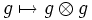# Group algebra as a Hopf algebra

## Definition

Let$G$ be a group and$K$ a field. The group algebra over$G$, when talked of as a Hopf algebra, is the following:

• The unital associative algebra part is the same as for the usual group algebra: We consider a vector space whose basis is indexed by elements of the group, and define multiplication of these basis elements by multiplication in the group.
• The comultiplication is defined by linearly extending the map:$g \mapsto g \otimes g$ for every$g$

In other words:$\nabla(\sum a_g g) = \sum a_g (g \otimes g)$

• The counit is defined by linearly extending the map:$g \mapsto 1$ for all$g$

In other words:$\epsilon(\sum a_g g) = \sum a_g$

• The antipode map is defined by linearly extending the map:$g \mapsto g^{-1}$

In other words:$S(\sum a_g g) = \sum a_g g^{-1}$